← Prev: Voltage and Current
→ Next: Ground

Ideal Sources

Ideal voltage and current sources are primitive concepts for modeling circuits. We discussed Voltage and Current in a previous section.

Here are the schematic symbols for these two ideal sources: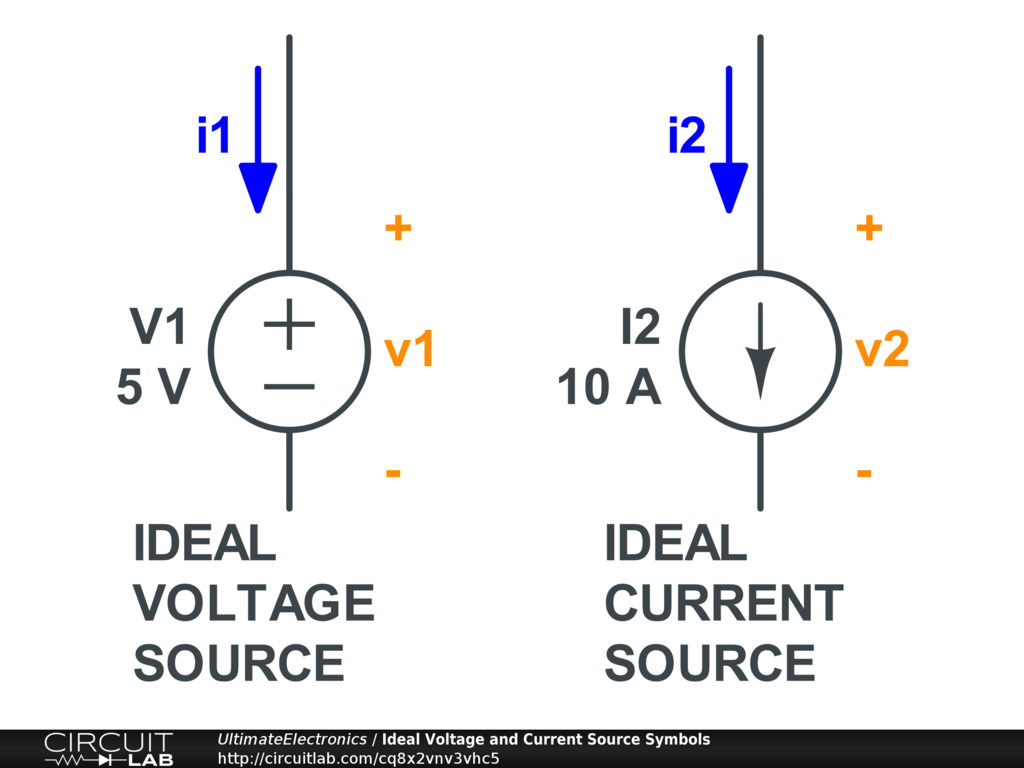Ideal Voltage Source

The symbol of the ideal voltage source is labeled with positive and negative terminals, indicating its direction. It's defined with just one parameter V: the voltage difference across its terminals.

The ideal voltage source maintains the same voltage difference across its terminals regardless of the magnitude of current, direction of current, or total charge delivered.

Ideal voltage sources do not exist in physical reality. A battery (an electrochemical cell) behaves sort of like an ideal voltage source, but this approximation breaks down when currents are large and/or when the battery is depleted. We'll discuss Batteries in more depth later.

When any current is pushed into or out of the ideal voltage source, the voltage across remains the same. Here's a simple simulation that shows this effect: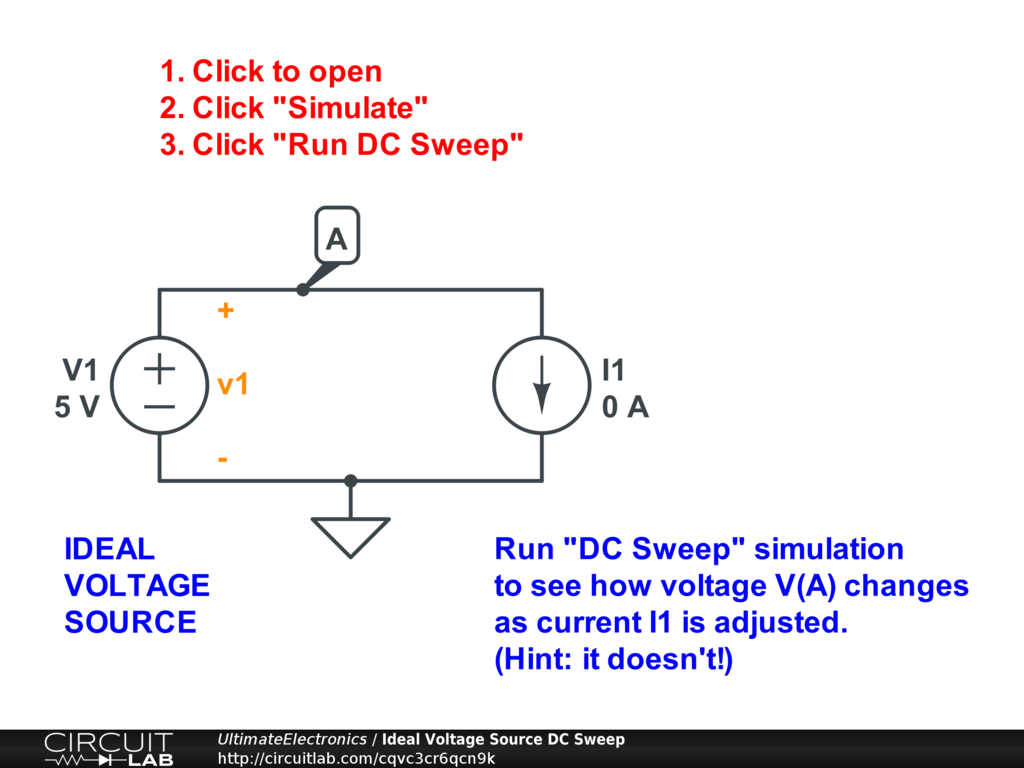Interactive Exercise Click the circuit, then click "Simulate," and "Run DC Sweep." It's set to adjust the current pushed into or out of the voltage source V1.

The voltage plot shows that the voltage remains constant (a flat line at 5 volts) regardless of the current. This is a boring simulation, but it shows the ideal voltage source doing exactly what it's supposed to!

Ideal Current Source

The symbol of an ideal current source has an arrow to indicate the direction of current -- assuming the defined current parameter I is positive.

The ideal current source pushes a certain constant amount of charge per time, regardless of voltage, energy, or total charge delivered.

A current source is also sometimes called a current sink, depending on which direction we're looking at it from. The term current source can be used in either direction.

There is no simple physical almost-matching component like the battery above. However, mechanical analogies exist. A water pump that always pushes out water at a predetermined constant rate, regardless of how hard it has to push against any obstructions in the pipe, is a reasonable hydraulic approximation to a current source.

This analogy hints at issues we'll see in electronics: what happens if we connect an ideal water pump with flow rate 1 liter/hour in series with an ideal water pump of flow rate 2 liters/hour? Which one wins? The answer is that something's got to give. Two ideal current sources in series are not compatible.

Similarly, what if we take our ideal water pump of 1 liter/hour and completely block its output pipe? This is like the same thing, because a blocked output is like a current source of 0 liters/hour. So, in the end, something's got to give -- either the pump or the obstruction -- but mathematically we've created an impossible situation.

Though they may not be found in nature, a current source is a valuable modeling technique because, over a fairly wide range, some things behave like current sources, and/or it's useful to build current sources as a subcomponent of other systems or models.

Here's a simple simulation showing that regardless of applied voltage, the current stays the same: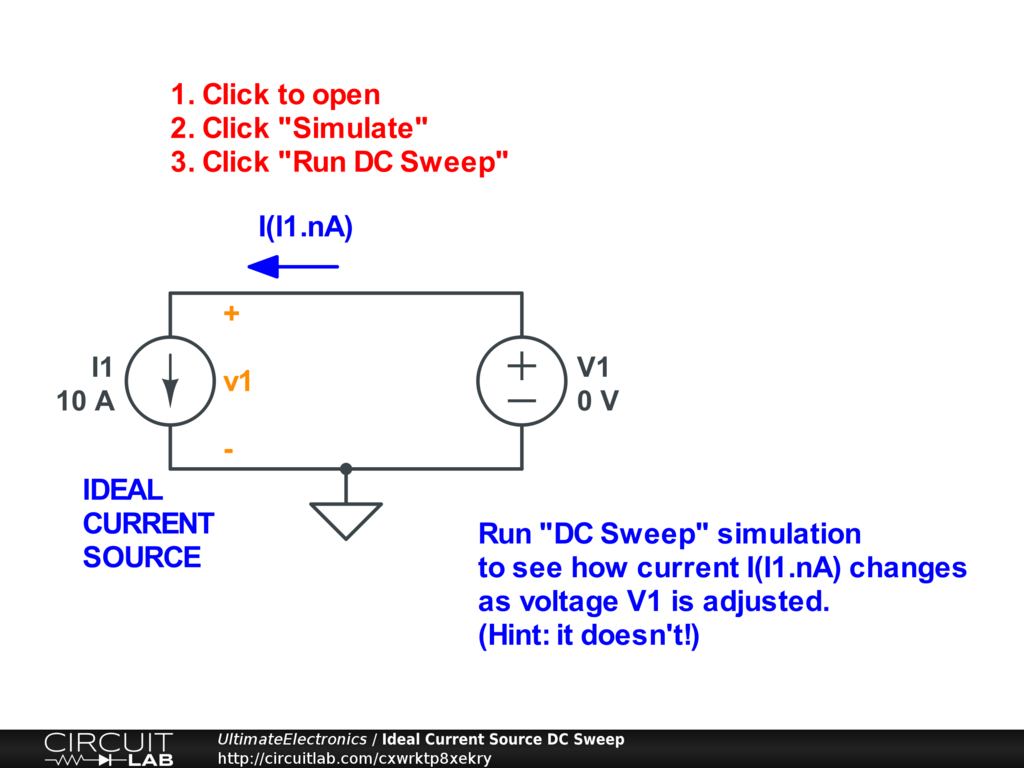Interactive Exercise Click the circuit, click "Simulate," and "Run DC Sweep."

Again, this is a boring flat-line simulation, but the current source keeps 10 Amps flowing regardless of the applied voltage.

Non-Ideal Sources

Modeling non-ideal sources requires thinking about Resistance and Ohm's Law as well as how voltages and currents behave in a network, as governed by Kirchhoff's Voltage Law and Kirchhoff's Current Law. However, these models are closely related to ideal sources, so we'll present them briefly here.

Non-Ideal (Practical) Voltage Source

A practical voltage source is modeled, to first order, with a series internal resistance: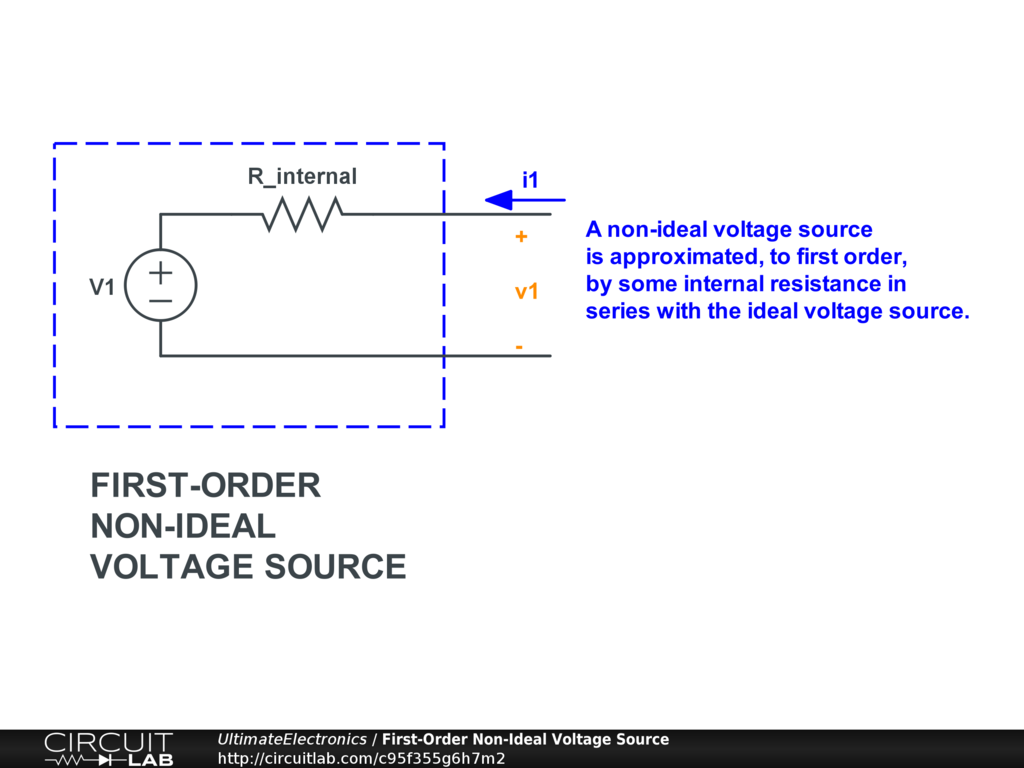This means that actual voltage seen externally drops as current is drawn from it. (Alternatively, the external voltage rises if current is pushed into it.)

Sometimes this effect is important, and sometimes it's not: it depends on the magnitude of the voltage drop and whether or not the rest of your system is designed to tolerate that.

In reality, it's not always linear. It's not even always monotonic: for example, look up overload crowbar protection to see how power supply designers sometimes intentionally want a nonlinear resistance behavior to protect a circuit from overloading and overheating.

Compare this non-ideal voltage source simulation to the one of the ideal voltage source above: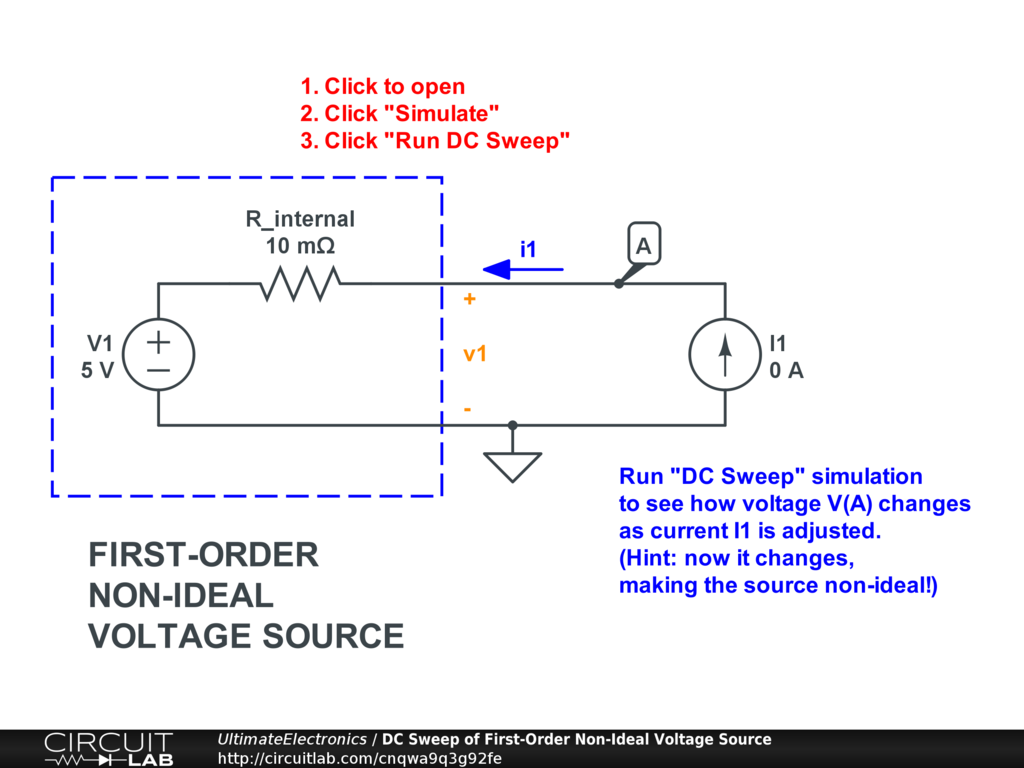Interactive Exercise Click the circuit, click "Simulate," and "Run DC Sweep." Now that there's a non-zero internal resistance, the voltage plot isn't flat anymore. The slope depends on the value of the internal resistance.

As batteries are often modeled as voltage sources, it's important to remember that real electrochemical battery cells do have internal resistance as well. This resistance depends on battery chemistry, construction, and history. Fresh, high-quality batteries will have lower internal resistance than old, used batteries. When people new to electronics consider driving a large load from batteries, they often forget to consider the voltage drop due to internal resistance, which can cause the system to not provide the expected power to the load. Additionally, the voltage drop can cause digital systems to reset, or can introduce power supply fluctuations on precision analog systems. Consider whether the internal battery resistance might affect the rest of your system.

Non-Ideal (Practical) Current Source

A practical current source is modeled, to first order, with a parallel internal resistance: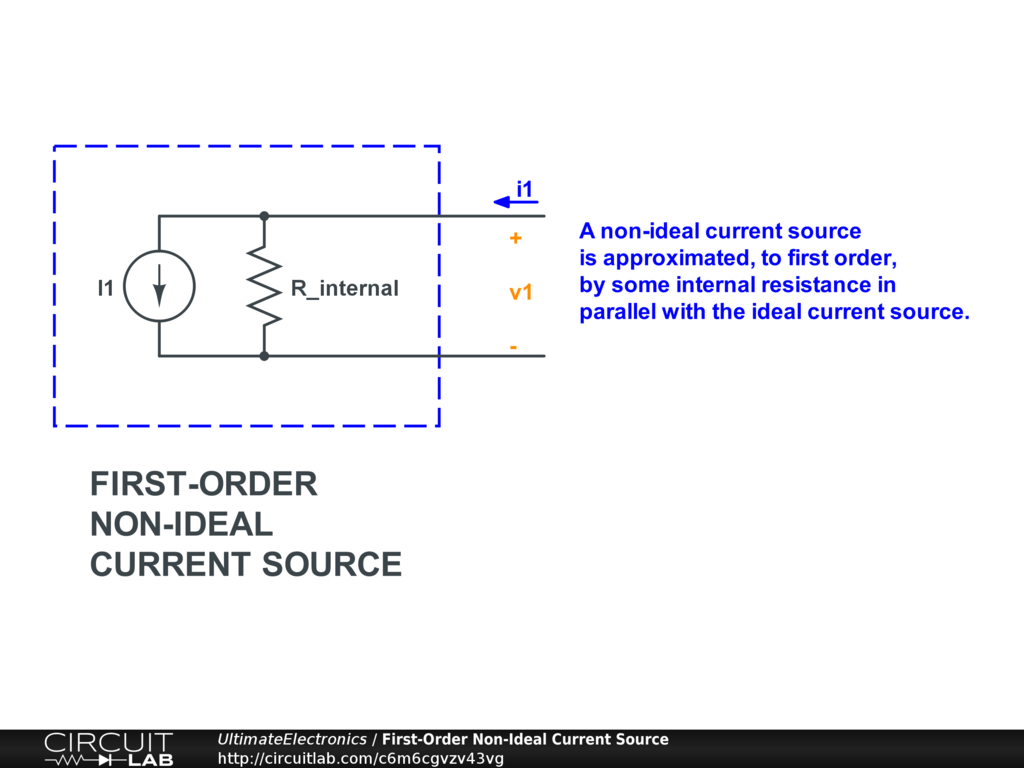Why is this extra resistor in parallel here, instead of in series like for the non-ideal voltage source? It's because a series resistor wouldn't do anything to an ideal current source. (A current source doesn't care about voltage drop, so any additional voltage drop due to the series resistor wouldn't affect the ideal source within.) Instead, a parallel resistor indicates that the current drawn will vary with applied voltage: both the ideal source and the resistor will draw current simultaneously.

Compare this non-ideal current source simulation to the one of the ideal current source above: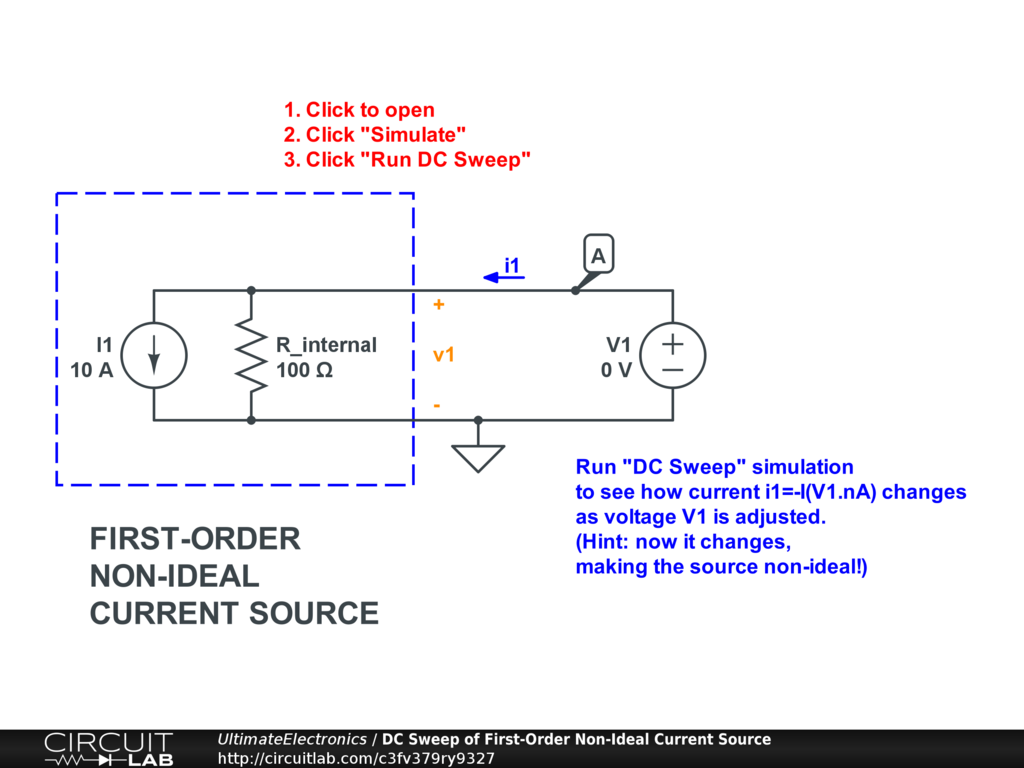Interactive Exercise Click the circuit, click "Simulate," and "Run DC Sweep." Now that there's a finite (no longer infinite) internal resistance, the current plot isn't flat anymore with external applied voltage.

Three Non-Ideal Modeling Choices

As there are no true ideal voltage and current sources is nature, a designer has three choices:

1. Model the entire nonlinear behavior of the source. This often has high complexity and is hard to understand.
2. Model the linearized behavior of the source near its operating point. This has much less complexity and is easier to understand.
3. Treat the nonideal source as being "close enough" to ideal (zero or infinite internal resistance). This has least complexity and is easiest to understand.

Practically speaking, this is a judgment that can and must be made by the engineer. Usually, modeling as #2 or #3 over some bounded range where we believe the effect is "flat enough to linearize" or "small enough to ignore" is a good call unless we're directly designing an intentionally nonlinear source.

The non-ideal voltage and current sources shown on this page are also called Thevenin Equivalent and Norton Equivalent Circuits, which we'll study in a later section.

What's Next

In the next section, Ground, we'll talk about the concept of a single zero voltage reference point -- a concept that's both incredibly widely used and incredibly confusing to many beginners.